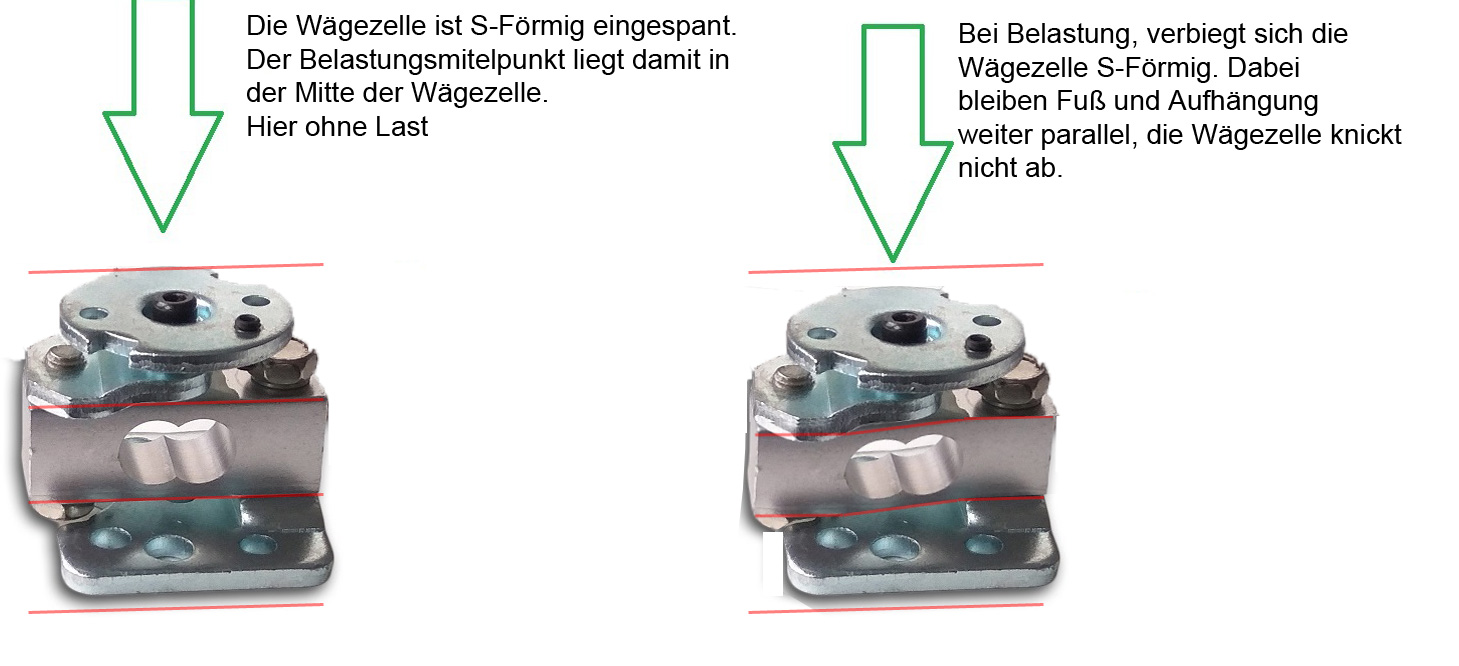# Tolerances of digital scales

2021-04-30 13:22:00 / / Comments 0

Note: The tolerance of a balance is usually described as "x d". "1 d" stands for 1 readability step on a scale, or "3 d" for 3 readability steps. For scales that have several measuring ranges and, for example, work below 10kg with 1g steps, above 10kg with 2g steps, a deviation of + - 5d would be either + - 5g (5 * 1g) or + - 10g (5 * 2g) during the measurement of larger weights.

In the case of balances, tolerances that occur under normal conditions are divided into the following areas:

1. Reproducibility

If you place the same weight several times in a row, the spread of the display must not exceed this value.
As an example: On a scale with 100g / 0.01g and a specified reproducibility of + - 1d, if you place an item that was measured as 10,00g may also be shown as 9.99g or 10.01g when it is lifted and placed again on the scale.

2. Linearity

Imagine the ideal measuring curve of a scale as a straight line, this is shown in the sketch with the black line. At the very bottom is the "0" point, which means that there is no weight on the weighing surface. At the very top right is the point of maximum capacity, at which the scale is loaded with e.g. 100g and at which the load cell pushes through by 1mm. This deformation is the normal value for most scales that work with a load cell based on strain gauges. If the balance is loaded with 50g, the load cell would have to push through by 0.5mm, with 25g by 0.25mm, etc ...This one millimeter deformation is the normal working range of a load cell that works with strain gauges, even if the scale is not designed up to 100g but up to 100kg. In this case, more force is required to deform the load cell over the same measuring range.

It also makes no difference whether the scales work up to 100g with 1g steps or up to 100g with 0.001g steps, both deform about the same amount under maximum load. For the 100g / 1g scale this means that the one millimeter is divided into 100 measuring steps and the scale has to recognize a new "measuring step" every 0.01 mm.
With a scale that can recognize 100g with 0.001g steps, this means that the areas between the steps are significantly smaller, now a new measuring step is output every 0.00001 mm.
The load cell must be of a much higher quality in order to deformation correctly at the entire measuring range.

This is where the problem begins: Depending on the material, there can always be points in the load cell where it yields a little more or less under different loads, i.e. with an actual 80g applied mass, it does not push through by 0.80000 mm, but by 0, 80050mm. On a scale that determines the weight in 100,000 measuring steps over this one millimeter and shows the weight every 0.001g, this results in a measuring error of 50 d.

The linearity is determined from a series of measurements made from weights that are steadily increasing in weight. The point at which the balance deviates the most is determined as and the point of maximum deviation is specified as "linearity", for example as + - 2 readability steps (= 0.2g for a balance that works with 0.1g steps).But: Please note that each scale can only work as accurate as the local conditions allow it to work.
Influences from the environment conditions like temperature, a yielding surface on which the scale is placed and air movements, inevitably lead to a change in the measurement signal.